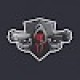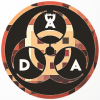# Floating Text+ (Addon) Update

If you want to give a personal touch to the world with floating writings, this addon is for you, like in real servers, you can go through it and the writing stays there, without it moving.With the new update you can rename and spawn with article.

=•=•=•=•=•=•=•=•=•=•=•=•=•=•=•=•=•=•=•=•=•=•=•=•=•=•=•=•=•=•=•=•=•=•=•==•=•=•=•=•=•=•=

To create the floating lettering is very simple.

First you need to spawn the entity.

-Doing / summon entity: floating_text text ~ ~ ~ name "Name that will be visible floating".

-For the second method just use floating text item

once you get the item rename it in the anvil, now you can do it with multiple lines.

once done place it on the ground with the right click.

If you once wrote and placed it, but you want to update it, just take the plate and rename it and then right click on the writing and it will change.

if you want to remove the holographic wording, just go over it and kill @e [r=1,type=entity: floating_text] and then remove it.

-Instead if you want to create written like this:

=•=•=•=•=•=•=•=•=•=•=•=•=•=•=•=•=•=•=•=•=•=•=•=•=•=•=•=•=•=•=•=•=•=•=•==•=•=•=•=•=•=•=

-To modify or use this package ask the creator.

-Don’t use the package as the creator, but give credit to its creator.

-Do not post the package on other sites.If you are caught using the package without consent, making changes or pretending to be your own you are against the copyright of the product provided for you and your enjoyment

=•=•=•=•=•=•=•=•=•=•=•=•=•=•=•=•=•=•=•=•=•=•=•=•=•=•=•=•=•=•=•=•=•=•=•==•=•=•=•=•=•=•=

Select version for changelog:

Changelog
the update consists in the addition of an item that allows you to spawn the writing and once done also to modify it.Update V 2.0.2 new item

### Installation

=•=•=•=•=•=•=•=•=•=•=•=•=•=•=•=•=•=•=•=•=•=•=•=•=•=•=•=•=•=•=•=•=•=•=•==•=•=•=•=•=•=•=

To install the pack:

Windows:Click on the downloaded file and start the installation automatically.

Android:You must have a File Manager, for example Rs File Manager. Once you have downloaded the texture, extract it in the games / com.mojang / resource_packs folder.

Console:You import it through the realms.

=•=•=•=•=•=•=•=•=•=•=•=•=•=•=•=•=•=•=•=•=•=•=•=•=•=•=•=•=•=•=•=•=•=•=•==•=•=•=•=•=•=•=

### Supported Minecraft versions

#### 14 Responses

Comments 14
4.6 / 5 (5 votes)11
What a cool idea!!
Log in to Reply1
What a cool idea!!!
Log in to Reply1
Thank you for making this forgot to put a rating on my last review
Log in to Reply1
imagine no problem, but if you have any suggestions, they are welcome.
Log in to Reply1
omg thank you so much for this
Log in to Reply1
using \n you can do a new line for the name, so it should be possible to do it without universal minecraft editor
Log in to Reply1
and i have a few questions with the rules- im assuming they translate to: if you are using the addon for a public server- ask the creator and credit the creator, do not steal the addon and do not reupload the addon without consent? Alot of the wording is off so its hard to get the context (not trying to sound mean)
Log in to Reply1
I should fix it, I know, but that's the point.
Not to steal the package and publish it on other sites, saying that you created it. If you have any advice I accept them.
Log in to Reply1
where would you type \n?
Log in to Reply1
I like the addon SO MUCh becase it makes my server a better look and i waited so long for this. THANK YOU SO MUCH
Log in to Reply1
Thank you, I'm very happy that you like our pack, also try the previous one Hud Actionbar +, it will give a touch to your server, if you have any ideas, feed it and we'll create it.
Log in to Reply1
Now that /playanimation + npc we can make floating vanilla text that won't disappear. But cool anyways.
Log in to Reply1
I know the method and that's why I created this addon, because it still has its flaws qul method, that every time you make a command block and if you want to make it float you use the tp, you avoid all this with this pack, it weighs nothing and does his duty, without overloading the world.
Log in to Reply